Trending Today#### Fidelity Bank: Stronger Price-Book Value, Needs To Watch Earnings, Profit Margin, NPL RatioCompanyFidelity Bank Plc (FIDELITYBK)
RatingHold
Current Market PriceN2.00
NPL RATIO (Q3-2019)6.53%
Year HighN2.90
Year LowN1.40
Fair ValueN2.34
Equity AnalystTunde Segun Jeariogbe

Introduction

• This report observed the financial performance of Fidelity Bank Plc for the first nine months of 2019, compared the same with the similar period in 2018 and established growth accordingly.
• We also combined a few valuation metrics to achieve conservative projections, using the full-year performance indices for the year ended 31st December 2019.
• Generally, the result can be rated average, when put side-by-side with figures released in the similar quarter of 2018.
• Non-performing loan ratio for the period jumped from 5.39% estimated in the 2019 half-year to 6.53%.
• Performance indices recorded an appreciable gap from the comparable periods, while Total Comprehensive Income improved substantially over a comparable period of 2018.

See below for other financial indices: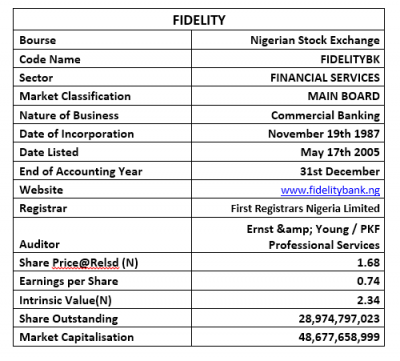Company figures

• Gross Earnings for the period stood at N161.05 billion up from the N139.00 billion posted at the end of the 2018 nine-month period.
• Interest Income stood at N135.11 billion, as against the N120.39 billion in the 2018 third-quarter.
• Interest Expenses was estimated at N76.87 billion, same as 23.52% over the reported N62.23 billion in 2018.
• Net Interest Income was estimated at N58.24 billion, as against the N58.16 billion achieved last year.
• Operating Expenses improved by 15.04% above the comparable period, as it is currently valued for N53.98 billion against N46.92 billion.
• Profit before Tax stood at N23.00 billion same as 14.65% above the N20.06 billion posted last year.
• Having considered the Tax Expense for the period, a total of N21.46 billion was reported as Profit for the period, which is 20.19% above the N17.85 billion earned at the end of the first nine months in 2018.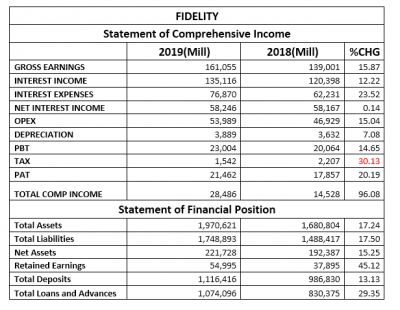• Total Assets is currently valued at N1.970 trillion, same as 17.24% above the corresponding period valuation of N1.68 trillion.
• Similarly, due to improved deposit, Total Liabilities posted for the period was N1.74 trillion, up from N1.48 trillion in 2018 third-quarter business session.
• Net Assets is therefore estimated at N221.72 billion, 15.25% above the N192.38 billion estimated last year.
• Retained Earnings remained positive at N54.99 billion against N37.89 billion last year.
• Total Deposit achieved at the end of the period is same as N1.11 trillion, higher than the N986.83 billion deposit reported in the corresponding quarter of 2018.
• Total Loans and Advances stood at N1.07 trillion against N830.37 billion in 2018

Financial Strength/Solvency Ratios

• Debt Ratio which compared both Total Assets to Total Liabilities stood at 0.89x or 89%, implying that the Total Liabilities is 89% of Total Assets. Understand that Total Liabilities is inclusive of Total Deposits for the period.
• Total Debt to Equity for the quarter stood at 7.89x, same as 1.95% above the 7.74x estimated in 2018. This implies that the company used Debt equivalent to 7.89 times of its equity through the period.
• Equity Ratio which compared Equity to Total Assets stood at 0.11x, same as corresponding quarter estimate; implying that the reported Equity at the end of the period is 11% of Total Assets.
• Estimated Beta value for Fidelity Bank as at the time this report was compiled stood at 1.57 this stood above-market beta the industrial average of 1.56.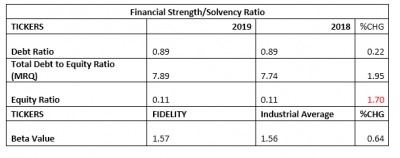Profitability Ratios

• Pre-Tax Margin dropped by a marginal 1.05% to 14.28%, as against the 14.43% estimated at the end of the 2018 nine-month business session.
• Interest Expenses to Gross Earnings inched by 6.61% to 47.73%, as against the previously estimated 44.77%.
• Return on Average Equity through the first nine months of 2019 was 9.68%, against 9.28% estimated from 2018 numbers.
• See the table below for detailed profitability ratios and comparison: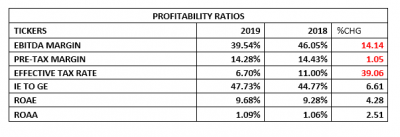Efficiency Ratios

• Operating Expenses figure posted at the end of the period is estimated at 33.52% of the estimated Gross Earnings, which is below the 33.76% achieved in the 2018 nine-month financials. This implies a marginal adjustment in the rate of expenses.
• Gross Earnings estimated from the bank’s financials is same as 8.17% of Total Assets value posted during the period under analysis.
• Total Loan and Advances posted for the quarter is 96.21% of Total Deposit, representing a 0.22% downward adjustment over the 84.15% estimate in 2018. Please note that this is far above the regulators’ standard for Nigerian banks.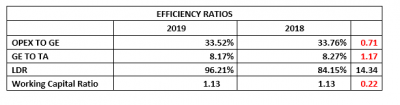Investment/Valuation Ratios

• Profit earned per unit of Fidelity Bank shares is estimated at N0.74, which is 20.19% above the N0.62 earned in the similar quarter of 2018.
• Total Comprehensive Income is estimated at N0.98, this is 96.08% above the N0.50 TCI/share achieved in 2018.
• Adjusted P/E-Ratio for nine months dropped to 0.76x from 1.08x, This can also be seen as the reduced waiting period for investors.
• The said earnings per share is a yielded 44.09% of the price of each unit of Fidelity Bank’s shares on the floor of the exchange on the day the result was unveiled to the investing public through the exchange.
• The estimated Book Value of each unit of Fidelity Bank is N7.65, this is 15.25% improvement over the previous estimate of N6.64 each.
• Confirming the academic under-priced nature of Fidelity Bank’s shares on the exchange is the Price to Book Value estimate that stood far below unity.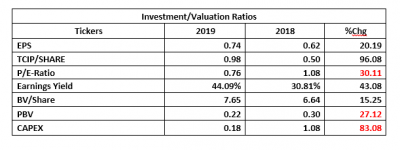Valuation

Exploring our blend of valuation tools, we conservatively valued each unit of Fidelity Bank shares at N2.34, thus, we rated it a Hold.

## Market Update